# RD Sharma Solutions Class 9 Maths Chapter 24 Measures Of Central Tendency Exercise 24.4

RD Sharma solutions Class 9 Chapter 24 is given here which consists of questions and answers related to Measure of Central Tendency. In this exercise, students will learn about the uses and properties of mode and median.

In a moderately symmetric distribution mean, median and mode are connected by the formule: Mode = 3 Median – 2 Mean.

Click on the link below to access all the solutions from RD Sharma class 9 .

## Download PDF of RD Sharma Solutions for Class 9 Maths Chapter 24 Measure of Central Tendency Exercise 24.4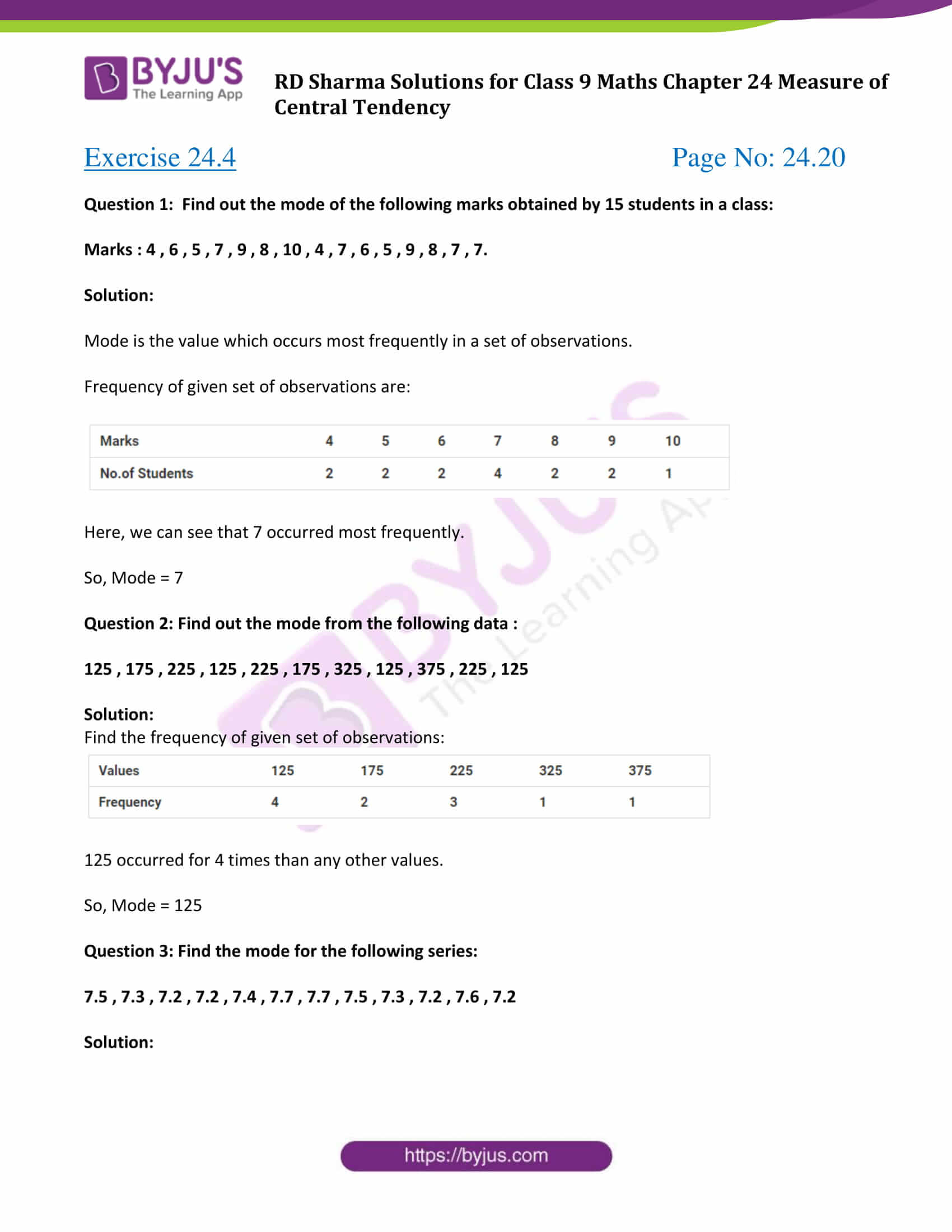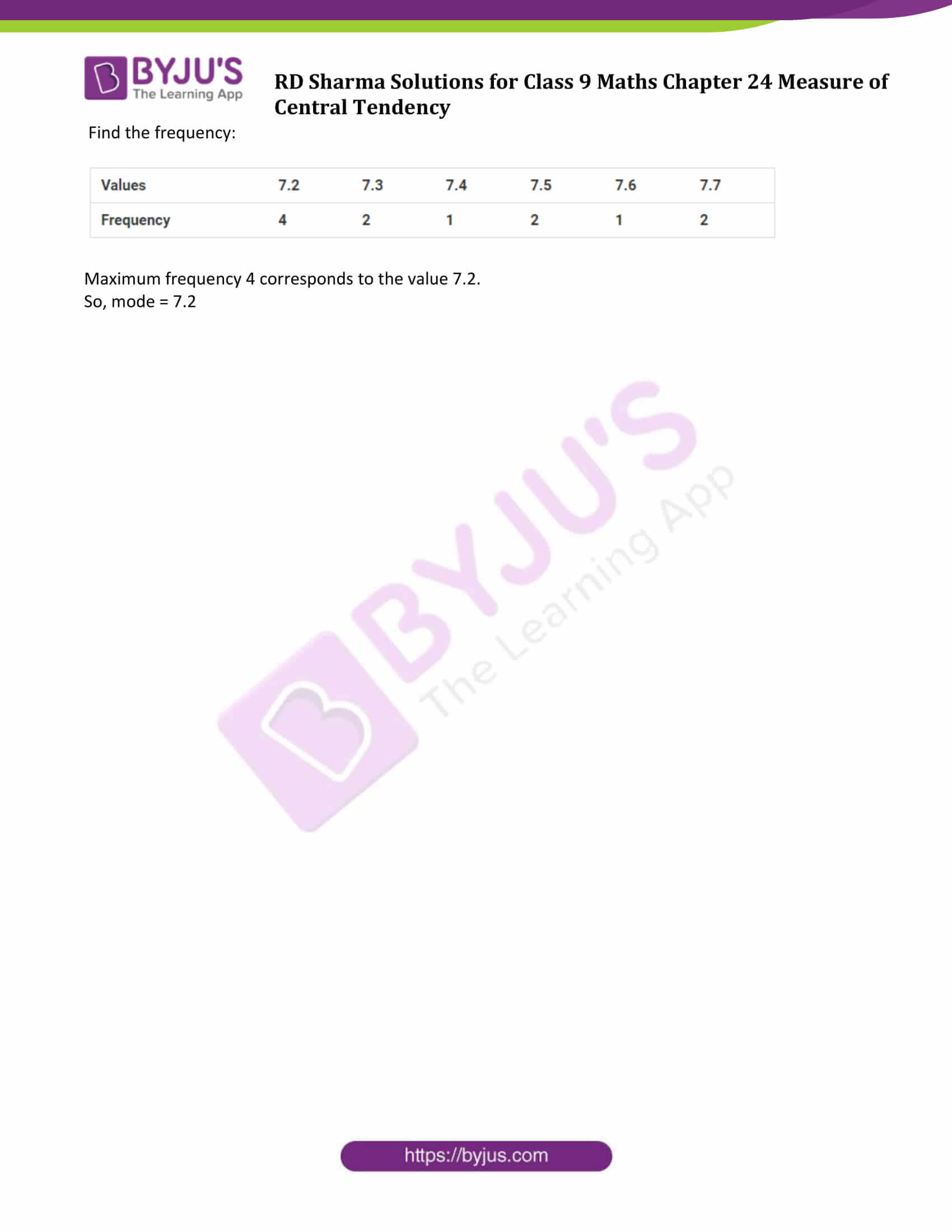### Access Answers to Maths RD Sharma Solutions for Class 9 Chapter 24 Measure of Central Tendency Exercise 24.4 Page number 24.20

Question 1: Find out the mode of the following marks obtained by 15 students in a class:

Marks : 4 , 6 , 5 , 7 , 9 , 8 , 10 , 4 , 7 , 6 , 5 , 9 , 8 , 7 , 7.

Solution:

Mode is the value which occurs most frequently in a set of observations.

Frequency of given set of observations are: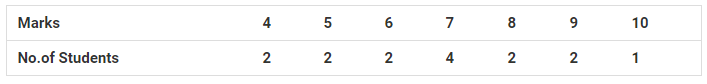Here, we can see that 7 occurred most frequently.

So, Mode = 7

Question 2: Find out the mode from the following data :

125 , 175 , 225 , 125 , 225 , 175 , 325 , 125 , 375 , 225 , 125

Solution:

Find the frequency of given set of observations: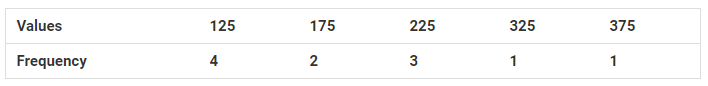125 occurred for 4 times than any other values.

So, Mode = 125

Question 3: Find the mode for the following series:

7.5 , 7.3 , 7.2 , 7.2 , 7.4 , 7.7 , 7.7 , 7.5 , 7.3 , 7.2 , 7.6 , 7.2

Solution:

Find the frequency: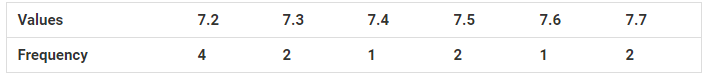Maximum frequency 4 corresponds to the value 7.2.

So, mode = 7.2

## RD Sharma Solutions for Class 9 Maths Chapter 24 Measures of Central Tendency Exercise 24.4

RD Sharma Solutions Class 9 Maths Chapter 24 Measures of Central Tendency Exercise 24.4 is based on the following topics:

• Uses of Median
• Properties of Median
• Mode
• Uses of Mode
• Properties of Mode